##### The Japanese Maple specialist
Direct order Contact Help / Services Newsletter# Shelf to displaySearch

Japanese MaplesYoung trees Acer seeds and more Bonsai soil and fertiliserVarieties introduced into EuropeHostasWind bells and chimesSaxifragesSpecial offer

Packing and shipping charges

# Beni komachi

› Japanese maples › Acer palmatum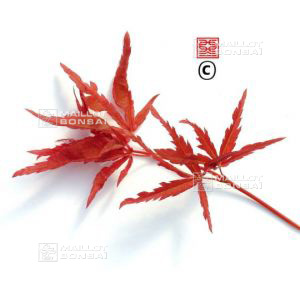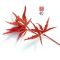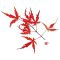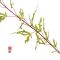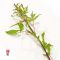ref. : 195

Choose the right size
Pot size

This item is temporarily unavailable

###### Description

Leaves: crinkled, reddish pink turning to brownish red during the summer and then marbled with brown in autumn. A delicate variety to grow, requiring protection from heavy rain in particular. Small leaves - 15/30 mm.

#palmatum 2.8 #komachi 2.7 #leaves 2.6 #acer 2.4 #beni 2.4 #protection 2 #particular 2 #requiring 1.9 #brownish 1.8 #crinkled 1.8

###### Technical description
 Species Acer palmatum same species items Variety Beni komachi Leaf colour Red leaves same colour items Leaf shape Palmated same shaped items Adult height Less than 2 meters same height items Shape Compact same shape items Exposure Sun same exposure items

Formule
(( ROUND((CHAR_LENGTH(b.article_nom)-CHAR_LENGTH(REPLACE(b.article_nom, 'komachi', '')))/LENGTH('komachi')) + ROUND((CHAR_LENGTH(b.article_description)-CHAR_LENGTH(REPLACE(b.article_description, 'komachi', '')))/LENGTH('komachi')) ) * 2.7) + (( ROUND((CHAR_LENGTH(b.article_nom)-CHAR_LENGTH(REPLACE(b.article_nom, 'leaves', '')))/LENGTH('leaves')) + ROUND((CHAR_LENGTH(b.article_description)-CHAR_LENGTH(REPLACE(b.article_description, 'leaves', '')))/LENGTH('leaves')) ) * 2.6) + (( ROUND((CHAR_LENGTH(b.article_nom)-CHAR_LENGTH(REPLACE(b.article_nom, 'beni', '')))/LENGTH('beni')) + ROUND((CHAR_LENGTH(b.article_description)-CHAR_LENGTH(REPLACE(b.article_description, 'beni', '')))/LENGTH('beni')) ) * 2.4) + (( ROUND((CHAR_LENGTH(b.article_nom)-CHAR_LENGTH(REPLACE(b.article_nom, 'protection', '')))/LENGTH('protection')) + ROUND((CHAR_LENGTH(b.article_description)-CHAR_LENGTH(REPLACE(b.article_description, 'protection', '')))/LENGTH('protection')) ) * 2) + (( ROUND((CHAR_LENGTH(b.article_nom)-CHAR_LENGTH(REPLACE(b.article_nom, 'particular', '')))/LENGTH('particular')) + ROUND((CHAR_LENGTH(b.article_description)-CHAR_LENGTH(REPLACE(b.article_description, 'particular', '')))/LENGTH('particular')) ) * 2) + (( ROUND((CHAR_LENGTH(b.article_nom)-CHAR_LENGTH(REPLACE(b.article_nom, 'requiring', '')))/LENGTH('requiring')) + ROUND((CHAR_LENGTH(b.article_description)-CHAR_LENGTH(REPLACE(b.article_description, 'requiring', '')))/LENGTH('requiring')) ) * 1.9) + (( ROUND((CHAR_LENGTH(b.article_nom)-CHAR_LENGTH(REPLACE(b.article_nom, 'brownish', '')))/LENGTH('brownish')) + ROUND((CHAR_LENGTH(b.article_description)-CHAR_LENGTH(REPLACE(b.article_description, 'brownish', '')))/LENGTH('brownish')) ) * 1.8) + (( ROUND((CHAR_LENGTH(b.article_nom)-CHAR_LENGTH(REPLACE(b.article_nom, 'crinkled', '')))/LENGTH('crinkled')) + ROUND((CHAR_LENGTH(b.article_description)-CHAR_LENGTH(REPLACE(b.article_description, 'crinkled', '')))/LENGTH('crinkled')) ) * 1.8) + (( ROUND((CHAR_LENGTH(b.article_nom)-CHAR_LENGTH(REPLACE(b.article_nom, 'delicate', '')))/LENGTH('delicate')) + ROUND((CHAR_LENGTH(b.article_description)-CHAR_LENGTH(REPLACE(b.article_description, 'delicate', '')))/LENGTH('delicate')) ) * 1.8) + (( ROUND((CHAR_LENGTH(b.article_nom)-CHAR_LENGTH(REPLACE(b.article_nom, 'marbled', '')))/LENGTH('marbled')) + ROUND((CHAR_LENGTH(b.article_description)-CHAR_LENGTH(REPLACE(b.article_description, 'marbled', '')))/LENGTH('marbled')) ) * 1.7)

## Secure payment## Delivery

Our logistic partners :04 74 55 23 48
Pépinière MAILLOT-BONSAÏ
Le Bois Frazy
01990 RELEVANT - FRANCE
on appointment